TensorSpace.js
Getting Start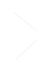Basic ConceptsTensorSpace ConverterModel PreprocessingModelsLayersMerge Function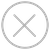Visualize pre-trained TensorFlow model using TensorSpace and TensorSpace-Converter
Introduction
In the following chapter, we will introduce the usage and workflow of visualizing TensorFlow model using TensorSpace and TensorSpace-Converter. In this tutorial, we will convert TensorFlow models with TensorSpace-Converter and visualize the converted models with TensorSpace.
This example uses LeNet trained with MNIST dataset. We provide pre-trained TensorFlow LeNet models for this example.
Sample Files
The sample files that are used in this tutorial are listed below:
filter_center_focus pre-trained TensorFlow models
Preprocess
First we will use TensorSpace-Converter to preprocess pre-trained different formats of TensorFlow models.
tf.keras model
For a tf.keras model, topology and weights can be saved in a HDF5 file xxx.h5. Use the following convert script:
``````\$ tensorspacejs_converter \
--input_model_from="tensorflow" \
--input_model_format="tf_keras" \
--output_layer_names="conv_1,maxpool_1,conv_2,maxpool_2,dense_1,dense_2,softmax" \
./rawModel/keras/tf_keras_model.h5 \
./convertedModel/layerModel``````
wb_sunnyNote:
• filter_center_focus Set input_model_from to be tensorflow.
• filter_center_focus Set input_model_format to be tf_keras.
• filter_center_focus Set .h5 file's path to positional argument input_path.
• filter_center_focus Get out the tf.keras layer names of model, and set to output_layer_names like Fig. 1.
• filter_center_focus TensorSpace-Converter will generate preprocessed model into convertedModel/layerModel folder, for tutorial propose, we have already generated a model which can be found in this folder.
tf.keras (separated)
For a tf.keras model, topology and weights can be saved in separate files, topology file xxx.json and weights file xxx.h5. Use the following convert script:
``````\$ tensorspacejs_converter \
--input_model_from="tensorflow" \
--input_model_format="tf_keras_separated" \
--output_layer_names="Conv2D_1,MaxPooling2D_1,Conv2D_2,MaxPooling2D_2,Dense_1,Dense_2,Softmax" \
./rawModel/keras_separated/topology.json,./rawModel/keras_separated/weights.h5 \
./convertedModel/layerModel``````
wb_sunnyNote:
• filter_center_focus Set input_model_from to be tensorflow.
• filter_center_focus Set input_model_format to be tf_keras_separated.
• filter_center_focus In this case, the model have two input files, merge two file's paths and separate them with comma (.json first, .h5 last), and then set the combined path to positional argument input_path.
• filter_center_focus Get out the tf.keras layer names of model, and set to output_layer_names like Fig. 1.
• filter_center_focus TensorSpace-Converter will generate preprocessed model into convertedModel/layerModel folder, for tutorial propose, we have already generated a model which can be found in this folder.
frozen model
For a TensorFlow frozen model. Use the following convert script:
``````\$ tensorspacejs_converter \
--input_model_from="tensorflow" \
--input_model_format="tf_frozen" \
--output_layer_names="MyConv2D_1,MyMaxPooling2D_1,MyConv2D_2,MyMaxPooling2D_2,MyDense_1,MyDense_2,MySoftMax" \
./rawModel/frozen/frozen_model.pb \
./convertedModel/graphModel``````
wb_sunnyNote:
• filter_center_focus Set input_model_from to be tensorflow.
• filter_center_focus Set input_model_format to be tf_frozen.
• filter_center_focus Set .pb file's path to positional argument input_path.
• filter_center_focus Get out the TensorFlow node names of model, and set to output_layer_names like Fig. 2.
• filter_center_focus TensorSpace-Converter will generate preprocessed model into convertedModel/graphModel folder, for tutorial propose, we have already generated a model which can be found in this folder.
saved model
For a TensorFlow saved model. Use the following convert script:
``````\$ tensorspacejs_converter \
--input_model_from="tensorflow" \
--input_model_format="tf_saved" \
--output_layer_names="MyConv2D_1,MyMaxPooling2D_1,MyConv2D_2,MyMaxPooling2D_2,MyDense_1,MyDense_2,MySoftMax" \
./rawModel/saved \
./convertedModel/graphModel``````
wb_sunnyNote:
• filter_center_focus Set input_model_from to be tensorflow.
• filter_center_focus Set input_model_format to be tf_saved.
• filter_center_focus Set saved model's folder path to positional argument input_path.
• filter_center_focus Get out the TensorFlow node names of model, and set to output_layer_names like Fig. 2.
• filter_center_focus TensorSpace-Converter will generate preprocessed model into convertedModel/graphModel folder, for tutorial propose, we have already generated a model which can be found in this folder.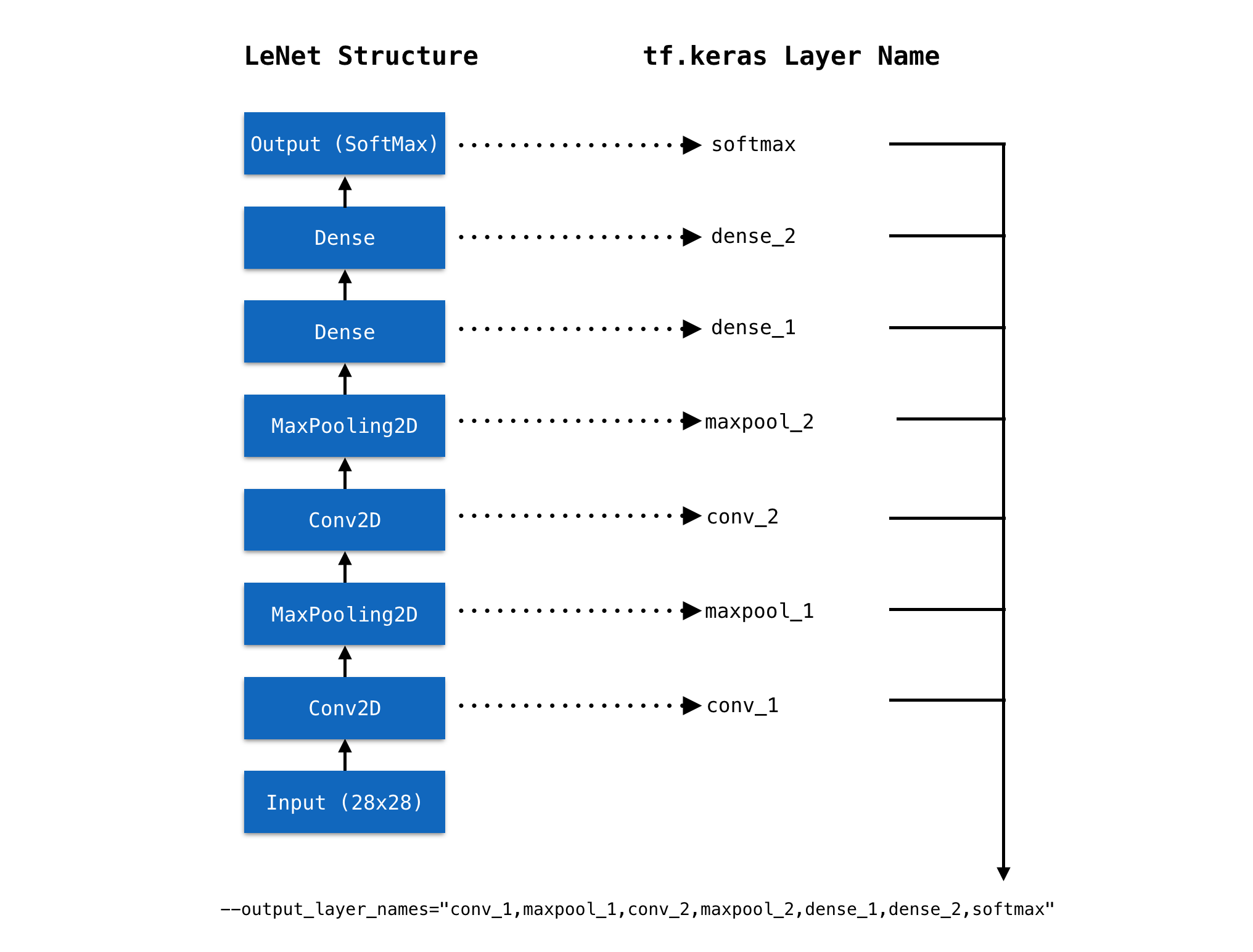Fig. 1 - Set tf.keras layer names to output_layer_names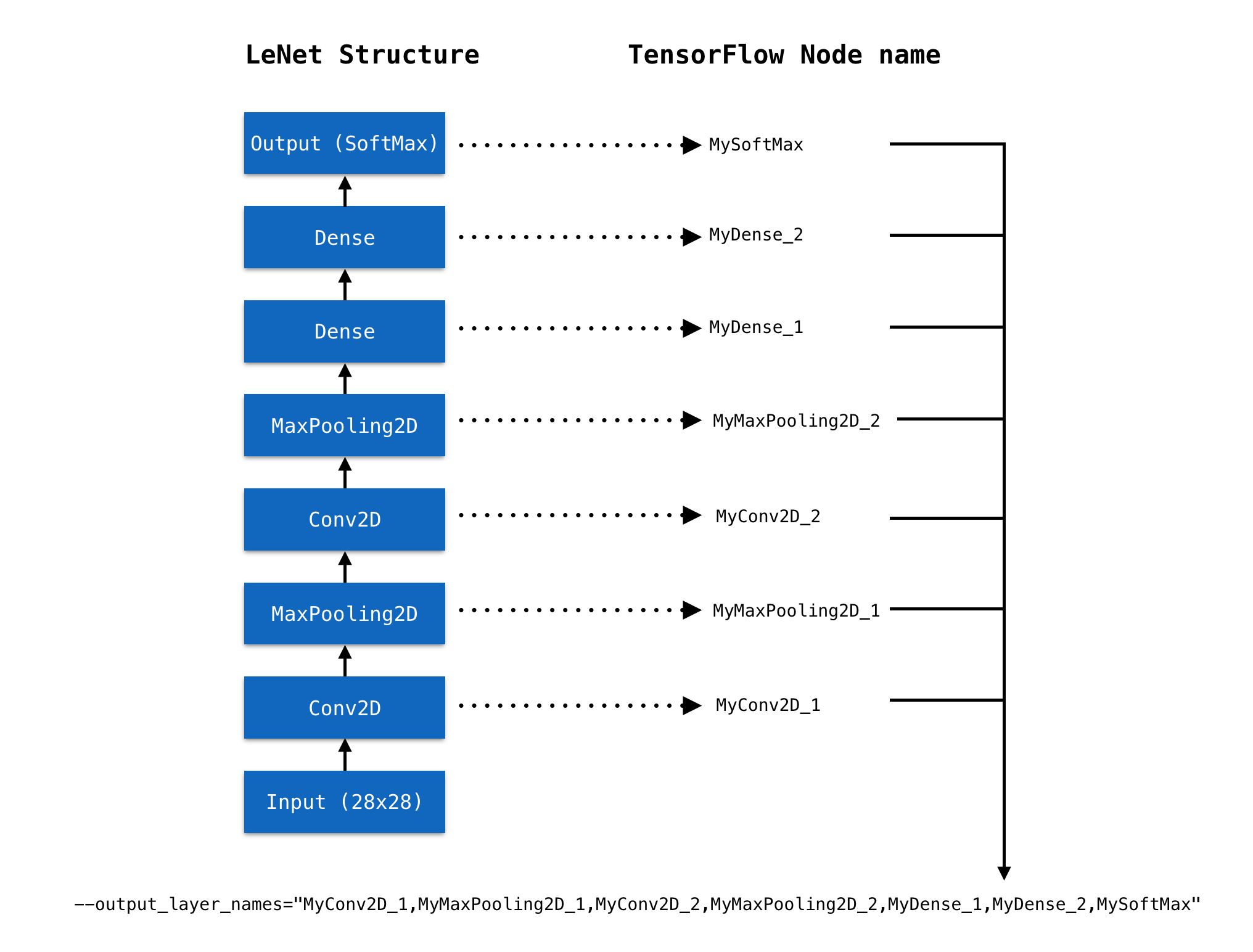Fig. 2 - Set TensorFlow node names to output_layer_names
After converting, we shall have the following preprocessed model:Fig. 3 - Preprocessed TensorFlow model
wb_sunnyNote:
• filter_center_focus There are two types of files created:
• flare One model.json file, describe the structure of our model (defined multiple outputs).
• flare Some weight files which contains trained weights. The number of weight files is dependent on the size and structure of the given model.
Then Apply TensorSpace API to construct visualization model.
``````let model = new TSP.models.Sequential( modelContainer );

outputs: [ "0", "1", "2", "3", "4", "5", "6", "7", "8", "9" ]
} ) );``````
For different kinds of TensorFlow models, configure TensorSpace Loader API in different ways.
filter_center_focus For layer model (tf.keras models) generated by TensorSpace-Converter:
``````model.load( {
type: "tensorflow",
url: "./convertedModel/layerModel/model.json"
} );``````
filter_center_focus For graph model (frozen model or saved model) generated by TensorSpace-Converter, it is required to configure outputsName, the same as the node names configured to output_layer_names in preprocessing:
``````model.load( {
type: "tensorflow",
url: "./convertedModel/graphModel/model.json",
outputsName: ["MyConv2D_1", "MyMaxPooling2D_1", "MyConv2D_2", "MyMaxPooling2D_2", "MyDense_1", "MyDense_2", "MySoftMax"]
} );``````
Then initialize the TensorSpace visualization model
``model.init();``
Result
If everything goes well, open the index.html file in browser, the model will display in the browser: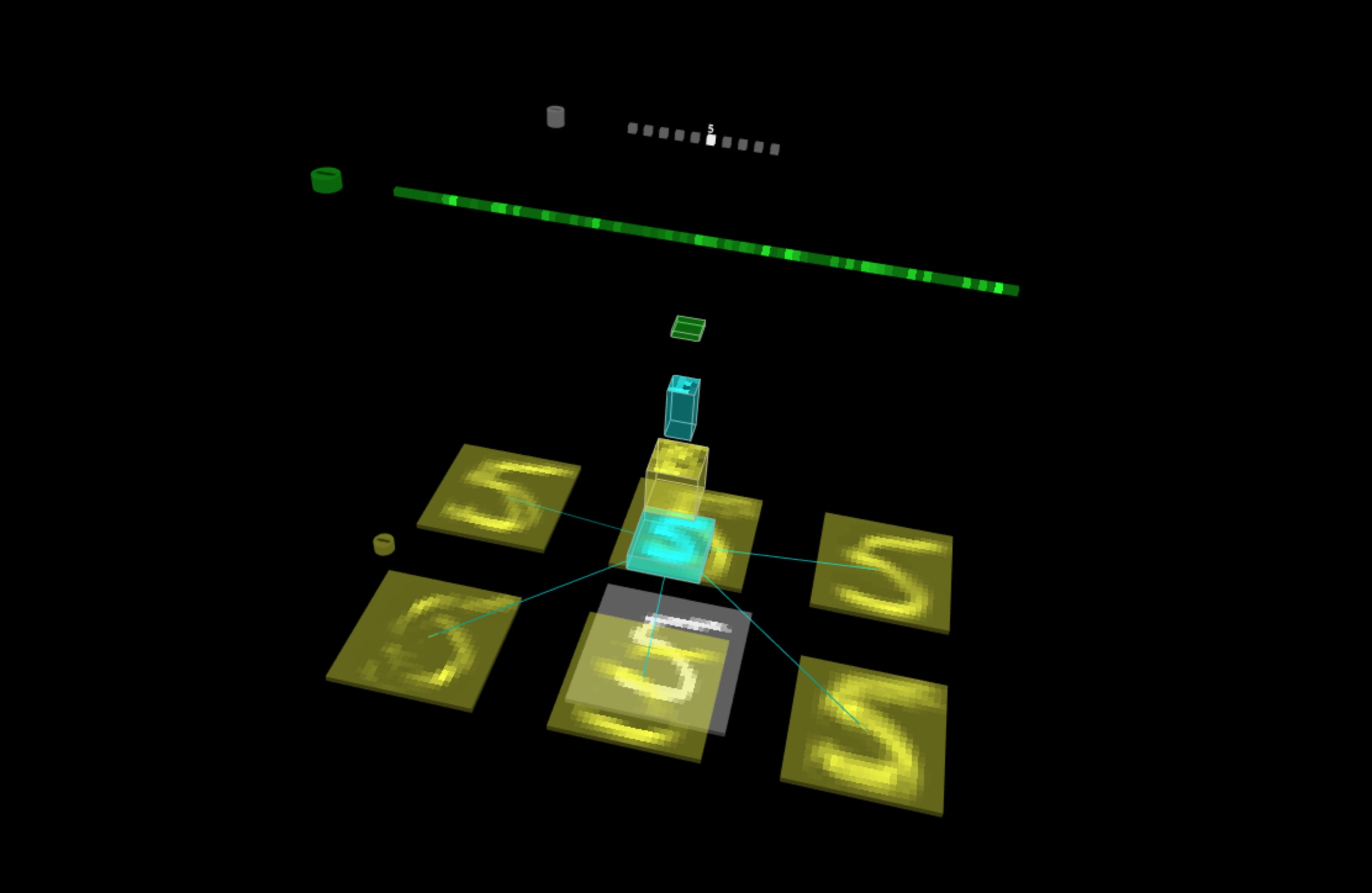Fig. 4 - TensorSpace LeNet with prediction data "5"